# Tables: Introduction & Solved Examples Notes | Study Logical Reasoning (LR) and Data Interpretation (DI) - CAT

## CAT: Tables: Introduction & Solved Examples Notes | Study Logical Reasoning (LR) and Data Interpretation (DI) - CAT

The document Tables: Introduction & Solved Examples Notes | Study Logical Reasoning (LR) and Data Interpretation (DI) - CAT is a part of the CAT Course Logical Reasoning (LR) and Data Interpretation (DI).
All you need of CAT at this link: CAT

What is a Table?

A table is a set of data arranged in rows and columns and is one of the most common ways of putting information across to people.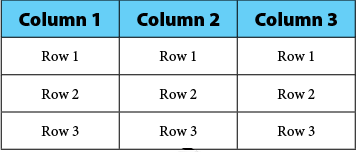A Table

• A table consists of several boxes with information inside. The first row and the first column are generally used to denote the titles.
• While any type of data can be presented in table form, that too in a very accurate manner, interpreting the data in table form is more difficult and time-consuming than the other modes, all of which are basically pictorial or graphical in presentation.
• Tables give values that are organized but not represented visually. You may graph the data from the table to make comparisons and see trends. You may create a table from a graph to make calculations easier.

Tips on Solving Table Chart Problems

1. Read the data very carefully, as the smallest detail may change the meaning of the question completely. Similarly, the instructions have to be understood carefully to prevent wasting time in calculating data that is not required, and also to find out exactly what is the answer that is sought.
2. Try to understand the data provided carefully, before jumping to answer the questions. The questions are designed to be deceptive, and a proper understanding of the requirements is a must. If the data provided is of the combined variety or if there are more than one data table / charts / graphs, try to understand the relation between the given tables.
Example: One table may talk about absolute sales figures, while the other table may talk of sales as a percentage of production. Hence, any question on excess production or Goods in stock will require data from both tables.
3. Be very careful of the units used in the tables, and the units in which the answers (options) are provided. A mistake in the units may yield an entirely different answer. Also, be careful of whether the answer is required in decimal or percentage. Such errors are common and easily avoidable.

Questions 1−4 are based on the table below, which is a record of the performance of a baseball team for the first seven weeks of the season.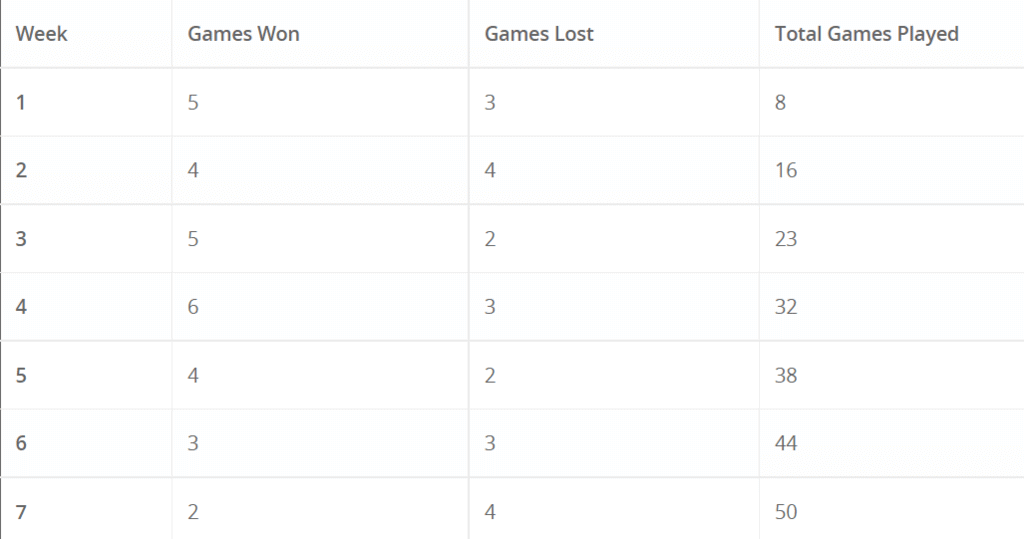Try yourself:How many games did the team win during the first seven weeks?

Q.2. What percent of the games did the team win?

(A) 21%

(B) 29%
(C) 42%
(D) 58%
(E) 72%

Solution:

The team won 29 out of 50 games.

29/50 = 58/100 = 58%

The correct answer is choice (D).

Try yourself:Which week was the worst for the team?

Q.4. Which week was the best week for the team?

(A) first week

(B) third week
(C) fourth week
(D) fifth week
(E) sixth week

Solution:

Compare the numbers in the win–loss columns. Since there is more than 1 week with more wins than losses, you need to do a quick calculation.

Method 1

Compare the values of the ratio wins/losses. The greatest value will be the best week.

5/3 ≈ 1.67

5/2 = 2.5

6/3 = 2

4/2 = 2

The value for the third week is 2.5.

The correct answer is choice (B).

Method 2

Find the percentage of games won using the number of wins and the number of games each week.

5/8 = 62.5%

5/7 ≈ 70%

6/9 ≈ 67%

4/6 ≈ 67%

The team won 70% of its games in the third week.

The correct answer is choice (B).

Example 1: Here is an example consisting of tabular data: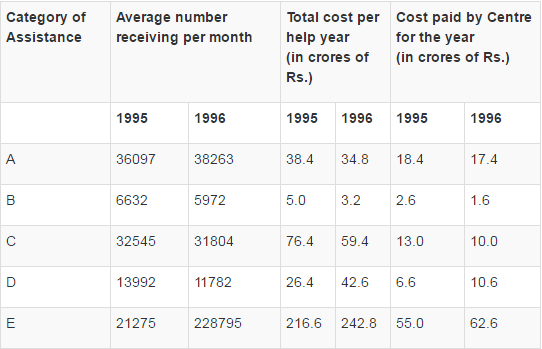Example 1.1: The category receiving the least percentage help from the centre (in the entire data) is:
(A) Category B in 1995
(B) Category C in 1996
(C) Category B in 1996
(D) Category D in 1995

• In this type of question, it is better to examine the alternatives given rather than trying to find the least percentage from the table.
• Let us now calculate the required percentage of the given alternatives: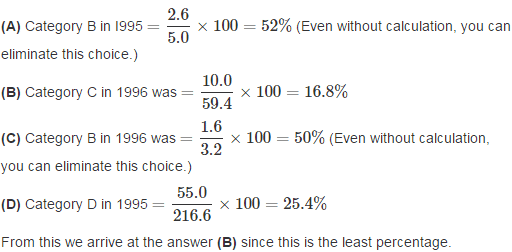Example 1.2: The difference between the average costs paid by the Centre during 1995 and 1996 is:
(A) Rs. 66 lakh
(B) Rs. 13.2 crore
(C) Rs. 132 lakh
(D) Rs. 13.2 lakh

#### Correct Answer is Option (C).

• Adding all the cost figures in the 1995 column, i.e.
18.4 + 2.6 + 13.0 + 6.6 + 55.0, you get 95.6.
• The average in 1995:
=95.6/Number of categories = 95.6/5
= Rs. 19.12
• Similarly, the average in 1996:
=(17.4+1.6+10.0+10.6+62.6) / 5
= Rs. 20.44
• The difference = Rs. (20.44−19.12)
= Rs. 1.32 cr
= Rs. 132 lakh
(Note how the answer needed conversion from crores to lakhs).

Example 1.3: Monthly cost to the city receiving E category assistance in 1996 is most nearly:
(A) Rs. 1.8 crore less than that in 1995
(B) Rs. 2.1 crore more than that in 1995
(C) Rs. 2.1 crore less than that in 1995
(D) Rs. 1.8 crore more than that in 1995

#### Correct Answer is Option (B).

• Here, straight calculation is only needed. We need to look at the total assistance figures.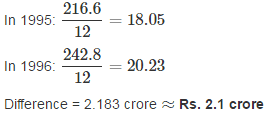Example 1.4: Assuming that 50% of the persons receiving category B help in 1995 were adults caring for minor children, but the city’s contribution towards maintaining these adults was 40% of the total contribution to B program in 1995, average amount paid by the city for each adult per year in 1995 is most nearly:
(A) Rs. 5900
(B) Rs. 6000
(C) Rs. 7500
(D) Rs. 3000.

#### Correct Answer is Option (B).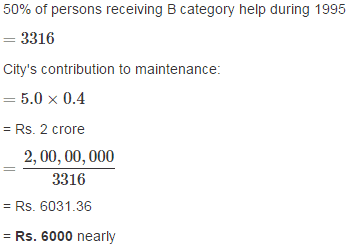Example 1.5: Monthly costs to the city of category D during 1995 and 1996 bear a ratio (most nearly):
(A) 2 : 3
(B) 5 : 3
(C) 3 : 2
(D) 3 : 5

#### Correct Answer is Option (D).

• Again, we can straightaway determine the answer through simple calculation.
• Since a ratio is required to be calculated, we can avoid the division by 12.
• Directly from the table we have, total assistance in 1995 and 1996 for Category D as 26.4 and 42.6.
• Hence the ratio is 26.4 : 42.6 = 3 : 5 nearly.

Example 2: Directions: Study the table carefully to answer the questions that follow.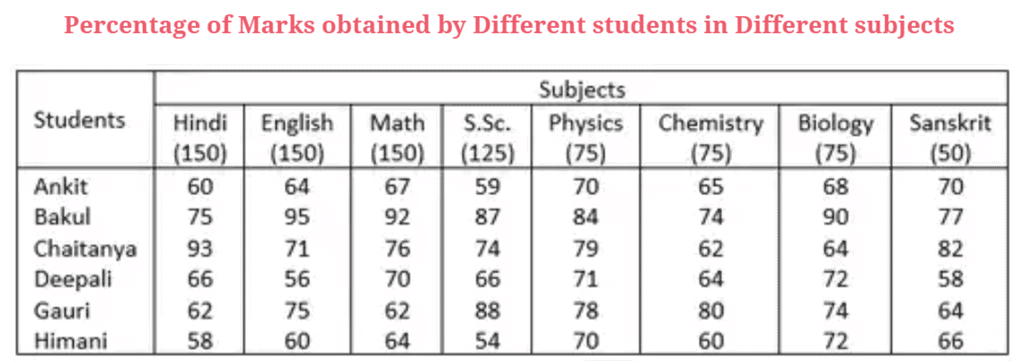Example 2.1: How many marks did Himani get in all the subjects together?

A. 505
B. 496
C. 525
D. 601
E. None of these

Solution: Required number = (58 ×150)/100 + (60 ×150)/100 + (64 ×150)/100 + (54 ×125)/100 + (70 ×75)/100 + (60 ×75)/100 + (72 ×75)/100 + (66 ×50)/100

= 87 + 90 + 96 + 67.5 + 52.5 + 45 + 54 + 33 = 525

Example 2.2: What are the average marks obtained by all students together in Physics?

A. 75.33
B. 56.5
C. 64.25
D. 48.88
E. None of these

Required average marks obtained = 452 × (75/100) × (1/6) = 56.5

Example 2.3: How many students have scored the highest marks in more than one subject?

A. Three
B. Two
C. One
D. None
E. None of these

Bakul, Chaitanya, and Gauri scored the highest marks in more than one subject.

Example 2.4: Marks obtained by Ankita in Sanskrit is what percent of marks obtained by Gauri in the same subject? (rounded off to two digits after decimal)

A. 91.43
B. 94.29
C. 103.13
D. 109.38
E. None of these

Required percentage = (70 × 100)/64 = 109.38

Example 2.5: Who has scored the highest marks in all the subjects together?

A. Chaitanya
B. Himani
C. Deepali
D. Gauri
E. None of those.

Total marks scored by Ankita = 90 + 96 + 100.5 + 73.75 + 52.5 + 48.75 + 51 + 35 = 547.5

Similarly

Total marks cored by Bakul=726.25

Total marks scored by Chaitanya = 647.25

Total marks scored by Deepali =554.75

Total marks scored by Gauri =614.5

Total marks scored by Himani = 525

Thus, highest marks are scored by Bakul.

The document Tables: Introduction & Solved Examples Notes | Study Logical Reasoning (LR) and Data Interpretation (DI) - CAT is a part of the CAT Course Logical Reasoning (LR) and Data Interpretation (DI).
All you need of CAT at this link: CATUse Code STAYHOME200 and get INR 200 additional OFF

## Logical Reasoning (LR) and Data Interpretation (DI)

55 videos|53 docs|102 tests

### How to Prepare for CAT

Read our guide to prepare for CAT which is created by Toppers & the best Teachers

Track your progress, build streaks, highlight & save important lessons and more!

,

,

,

,

,

,

,

,

,

,

,

,

,

,

,

,

,

,

,

,

,

;